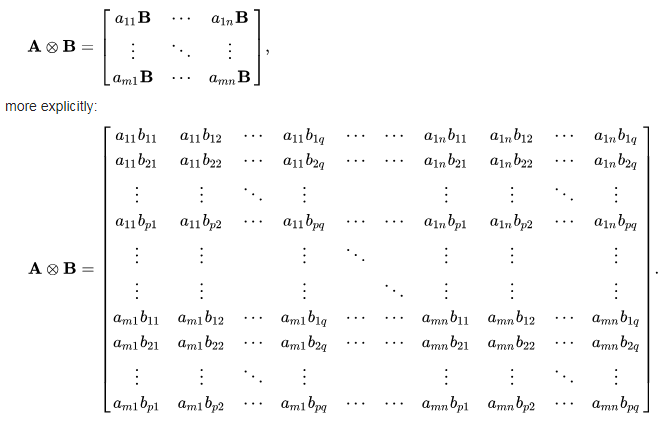﻿ NumPy: Compute the Kronecker product of two given mulitdimension arrays - w3resource# NumPy: Compute the Kronecker product of two given mulitdimension arrays

## NumPy: Linear Algebra Exercise-8 with Solution

Write a NumPy program to compute the Kronecker product of two given mulitdimension arrays.

Note: In mathematics, the Kronecker product, denoted by ⊗, is an operation on two matrices of arbitrary size resulting in a block matrix. It is a generalization of the outer product (which is denoted by the same symbol) from vectors to matrices, and gives the matrix of the tensor product with respect to a standard choice of basis. The Kronecker product should not be confused with the usual matrix multiplication, which is an entirely different operation.

If A is an m × n matrix and B is a p × q matrix, then the Kronecker product A ⊗ B is the mp × nq block matrix:Sample Solution :

Python Code :

``````import numpy as np
a = np.array([1,2,3])
b = np.array([0,1,0])
print("Original 1-d arrays:")
print(a)
print(b)
result =  np.kron(a, b)
print("Kronecker product of the said arrays:")
print(result)
x = np.arange(9).reshape(3, 3)
y = np.arange(3, 12).reshape(3, 3)
print("Original Higher dimension:")
print(x)
print(y)
result = np.kron(x, y)
print("Kronecker product  of the said arrays:")
print(result)
``````

Sample Output:

```Original 1-d arrays:
[1 2 3]
[0 1 0]
Kronecker product of the said arrays:
[0 1 0 0 2 0 0 3 0]
Original Higher dimension:
[[0 1 2]
[3 4 5]
[6 7 8]]
[[ 3  4  5]
[ 6  7  8]
[ 9 10 11]]
Kronecker product  of the said arrays:
[[ 0  0  0  3  4  5  6  8 10]
[ 0  0  0  6  7  8 12 14 16]
[ 0  0  0  9 10 11 18 20 22]
[ 9 12 15 12 16 20 15 20 25]
[18 21 24 24 28 32 30 35 40]
[27 30 33 36 40 44 45 50 55]
[18 24 30 21 28 35 24 32 40]
[36 42 48 42 49 56 48 56 64]
[54 60 66 63 70 77 72 80 88]]
```

Python Code Editor:

Have another way to solve this solution? Contribute your code (and comments) through Disqus.

What is the difficulty level of this exercise?

Test your Python skills with w3resource's quiz

﻿

## Python: Tips of the Day

Negative Indexing:

In Python you can use negative indexing. While positive index starts with 0, negative index starts with -1.

```name="Welcome"
print(name)
print(name[-1])
print(name[0:3])
print(name[-1:-4:-1])

```

Output:

```W
e
Wel
emo```